Next: Traveling Waves in an Up: Traveling Waves Previous: Traveling Waves

# Standing Waves in a Finite Continuous Medium

We saw earlier, in Sections 5.2 and 294, that a small amplitude transverse wave on a uniform string, and a small amplitude longitudinal wave on an elastic rod, are both governed by the wave equation, which (in one dimension) takes the general form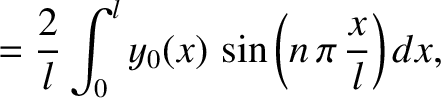(361)

where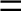represents the wave disturbance, and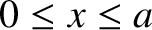is a constant, with the dimensions of velocity, that is a property of the particular medium that supports the wave. Up to now, we have only considered media of finite length. That is, media that extend from (say)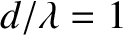to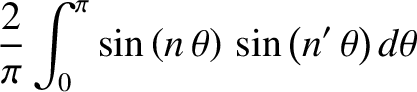. Generally speaking, we have encountered two distinct types of physical constraint that hold at the boundaries of such media. First, if a given boundary is fixed then the wave disturbance is constrained to be zero there. For instance, if the left boundary is fixed then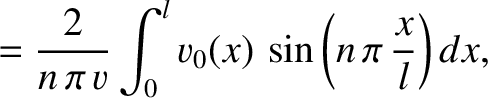. Second, if a given boundary is free then the spatial derivative of the disturbance (which usually corresponds to some sort of force) is constrained to be zero there. For instance, if the right boundary is free then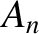. It follows that a fixed boundary corresponds to a node--that is, a point where the amplitude of the wave disturbance is always zero. On the other hand, a free boundary corresponds to an anti-node--that is, a point where the amplitude of the wave disturbance is always locally maximal. Consequently, the nodes and the anti-nodes of a wave, of definite wavelength, supported in a medium of finite length that has stationary boundaries (which can be either fixed or free) are constrained to be stationary. The only simple solution of the wave equation, (361), that has stationary nodes and anti-nodes is a standing wave of the general form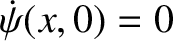(362)

The associated nodes are located at the values of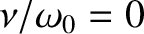that satisfy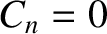(363)

which implies that they are stationary, and also evenly spaced a distance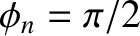apart, whereis the wavelength. Moreover, the anti-nodes are situated halfway between the nodes. For example, suppose that both boundaries of the medium are fixed. It follows that the pointsandmust each correspond to a node. This is only possible if the length of the medium,, is a half-integer number of wavelengths: that is,, where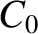is a positive integer. We conclude that, in this case, the possible wavenumbers of standing wave solutions to the wave equation are quantized such that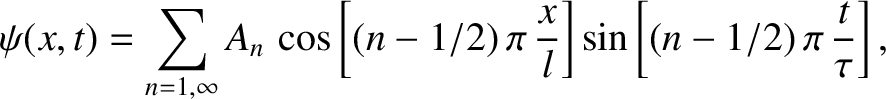(364)

Moreover, the same is true if both boundaries are free. Finally, if one boundary is free, and the other fixed, then the quantization of wavenumbers takes the slightly different form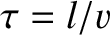(365)

Those standing wave solutions that satisfy the appropriate quantization criterion are known as the normal modes of the system. (See Chapter 4.) Moreover, substitution of Equation (362) into the wave equation, (361), yields the standing wave dispersion relation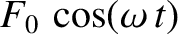(366)

The fact that normal mode wavenumbers are quantized immediately implies that the associated oscillation frequencies are also quantized. Finally, because the wave equation is linear, the most general solution that satisfies the boundary conditions is a linear superposition of all of the normal modes. Such a solution has the appropriate node or anti-node at each of the boundaries, but does not necessarily have any stationary nodes or anti-nodes in the interior of the medium.Next: Traveling Waves in an Up: Traveling Waves Previous: Traveling Waves
Richard Fitzpatrick 2013-04-08